# Descriptive Statistics

### Measures of Centrality & Dispersion

#### 2020-08-31

# clean workspace
rm(list=ls())
load("../data/data_qlfs.RData") 

# 2 Type of Data

Recall, there are two main types of data:

## 2.1 Categorical

Variable has response categories

Nominal: no specific order to the categories eg. gender (male/female)

Ordinal: categories have a clear ranking eg. age groups (young; middle aged; old)

## 2.2 Continuous (aka Scale)

Variable is a precise measure of a quantity.

Continuous (skewed): distribution of measures NOT symmetrical about the mean (skew <> 0) eg. income (to nearest penny)

Continuous (symmetrical): distribution of measures IS symmetrical about the mean (skew = 0) eg. height (to nearest mm)

The graphs below illustrate the difference between a symmetrical and a skewed distribution: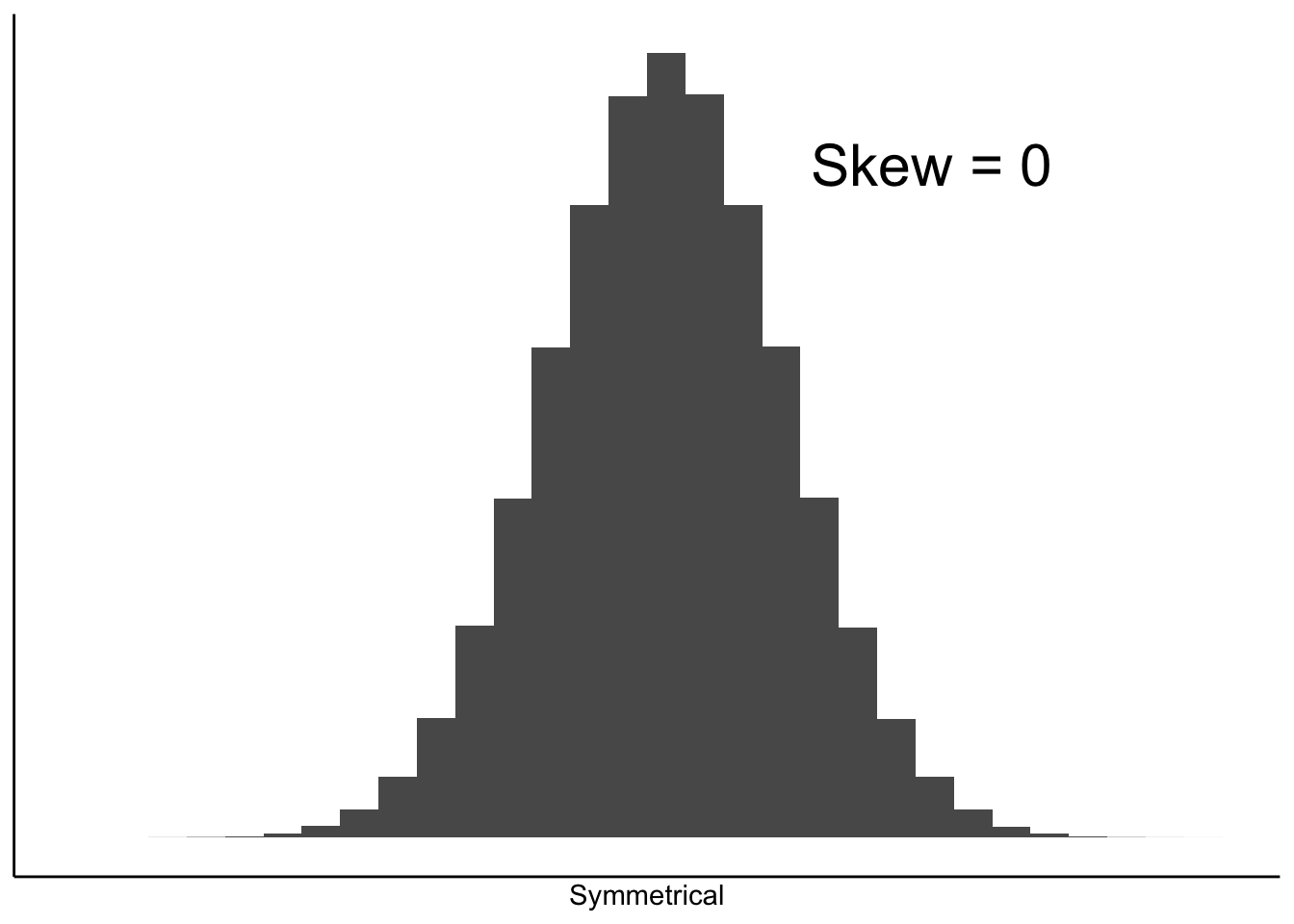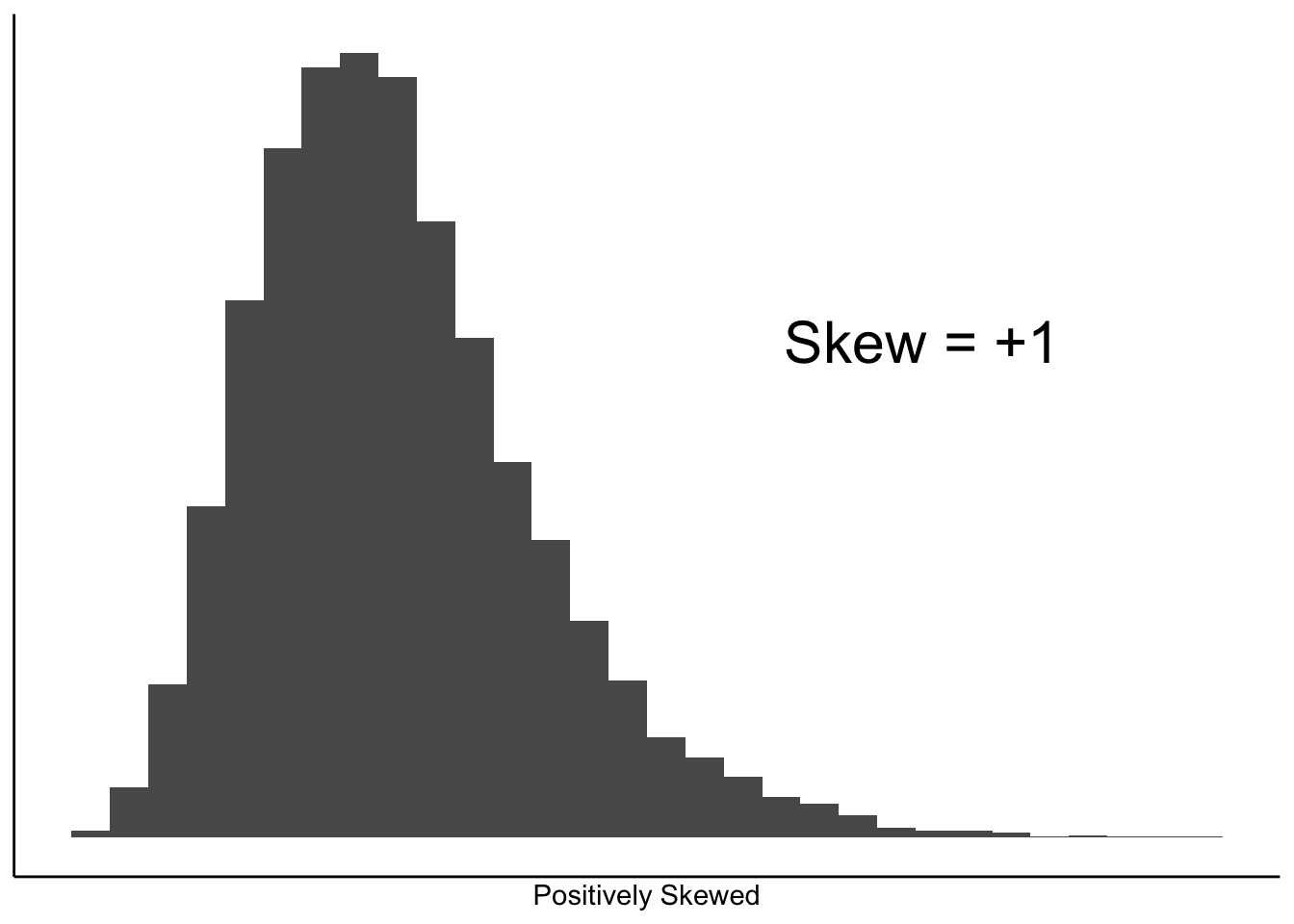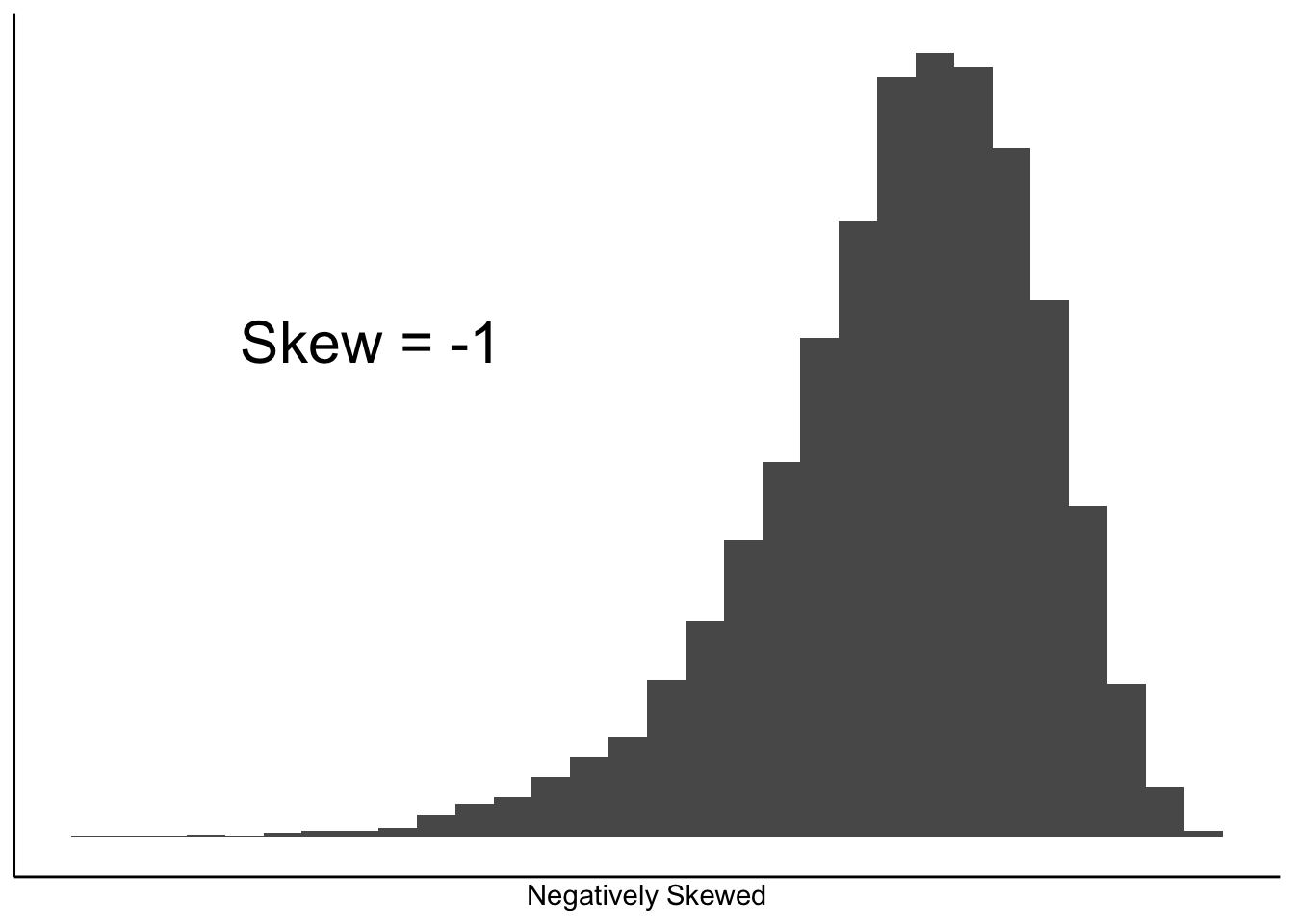Fig.1 Skewness of a distribution

TASK #1 Work out the data type for each of the following variables:

• Marital status (MaritalStatus)
• Age (Age)
• Age group (AgeGroup)
• Tenure (Tenure)
• Gross weekly pay (GrossPay)

# 3 Measures of Central Tendency

Central tendency is statistical jargon for ‘the average / mean’.

It is important to realise that the statistic used to measure the average varies by data type:

Data Type Measure of average
Nominal Mode
Ordinal Median
Scale (skewed1) Median
Scale (symmetrical1) Mean

1 Symmetrical = skew close to 0 (i.e. in range -0.5 to 0.5)

# attach data
attach(qlfs)

## 3.1 Mean

mean(Age)
##  46.08521

## 3.2 Media

median(Age)
##  46

## 3.3 Mode

R does not have a standard in-built function to calculate mode. So we create a user function to calculate mode of a data set in R.

## create the function.
mode <- function(v) {
uniqv <- unique(v)
uniqv[which.max(tabulate(match(v, uniqv)))]
}

mode(Age)
##  41

Or you can use:

summary(Age)
##    Min. 1st Qu.  Median    Mean 3rd Qu.    Max.
##   16.00   32.00   46.00   46.09   60.00   99.00

Or for all the variables in the data

summary(qlfs)

TASK #2 Calculate the most appropriate measure of the ‘average’ for each of the following variables:

• Marital status (MaritalStatus)
• Age group (AgeGroup)
• Tenure (Tenure)
• Gross weekly pay (GrossPay)

# 4 Measures of Dispersion

Dispersion is statistical jargon for the spread of a distribution.

The graphs below show two symmetrical distributions; one with a wide spread (large dispersion); and one with a narrow spread (small dispersion).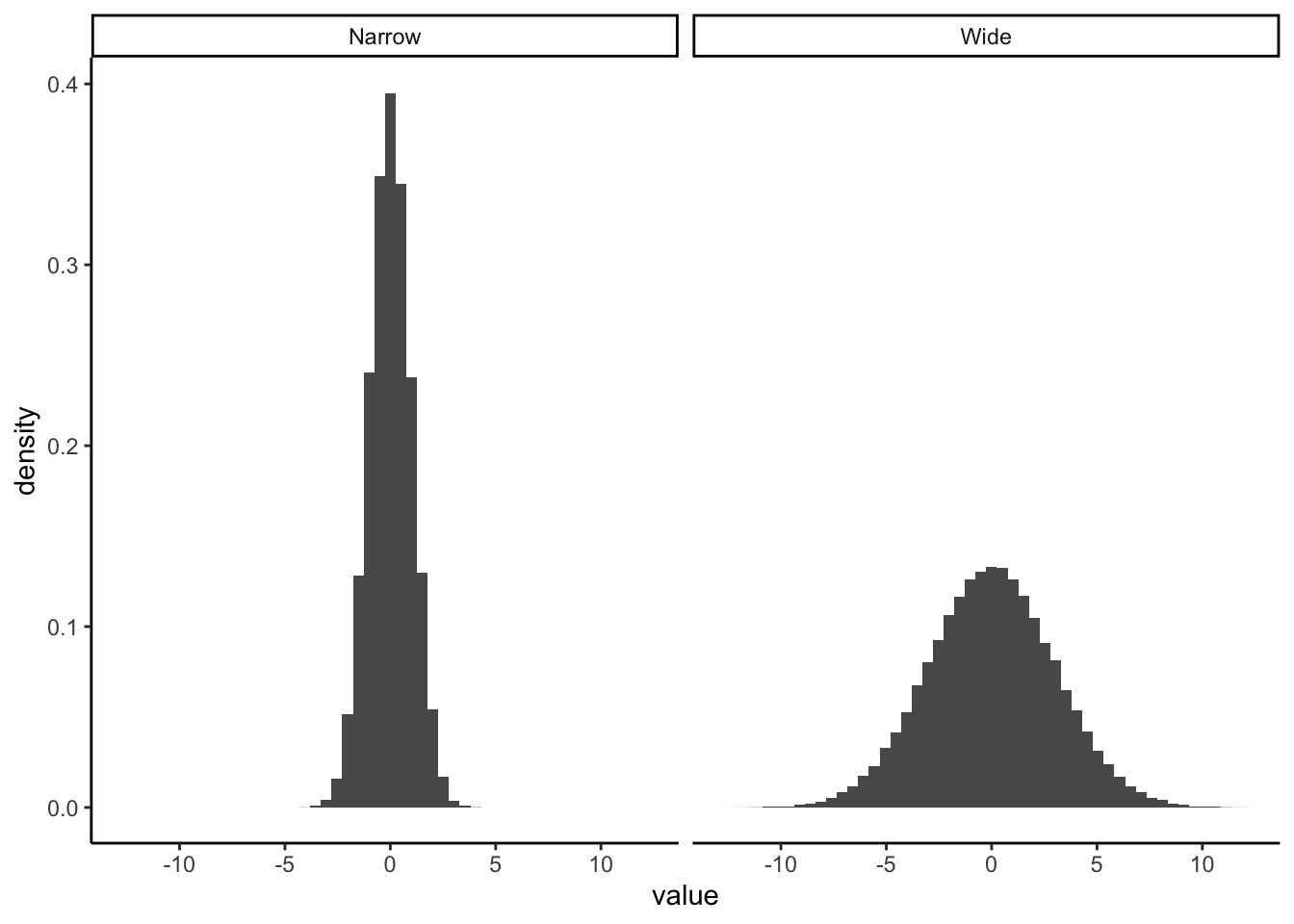Fig.2 Dispersion

The most appropriate statistic for summarising the dispersion (spread) of a distribution also varies by data type:

Data Type Measure of dispersion
Nominal % Misclassified
Ordinal % Misclassified
Scale (skewed) Inter-Quartile Range
Scale (symmetrical) Standard Deviation / Variance

## 4.1 Dispersion of Continuous Variables

### 4.1.1 Central Tendency As a Model of The Data

People and places are complex entities. The art of statistical analysis is to distil the essence of this complexity into simplified models of reality.

The average is the simplest model of all, and is widely used. We are frequently told that average wages, health or educational outcomes, sales have gone up or down.

This begs the immediate question: how well does our model represent the reality?

We can best illustrate this by focussing on only the first 10 cases in our data, and treating them as if they were the entire population (Fig. 3).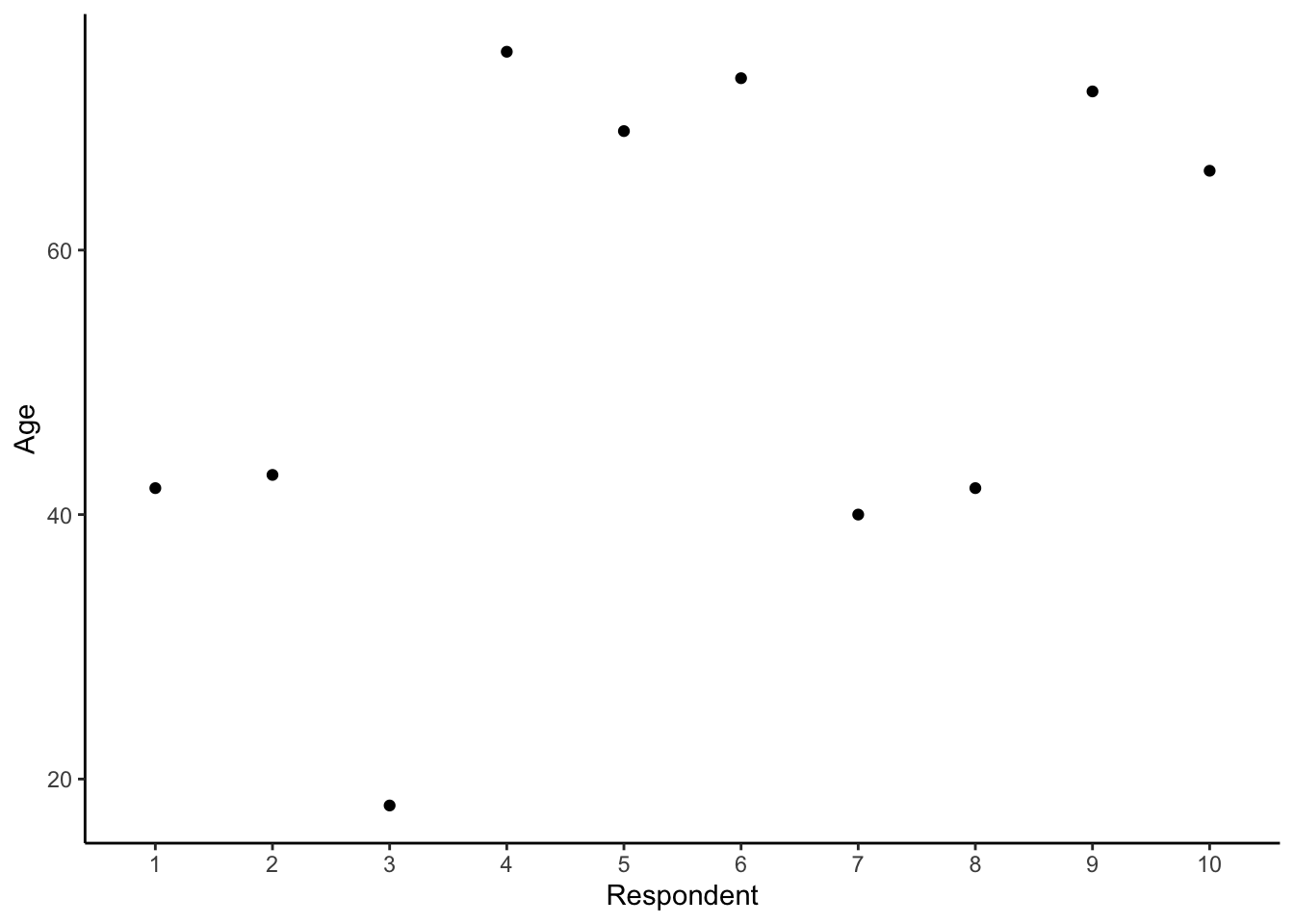Fig.3 Age of 10 respondents

As can be seen from this graph, the age of these 10 respondents varies from 18 to 75.

A simple model of respondent’s age is their average (mean) age.

We can add a dashed horizontal line to the graph to represent this model (Fig. 4).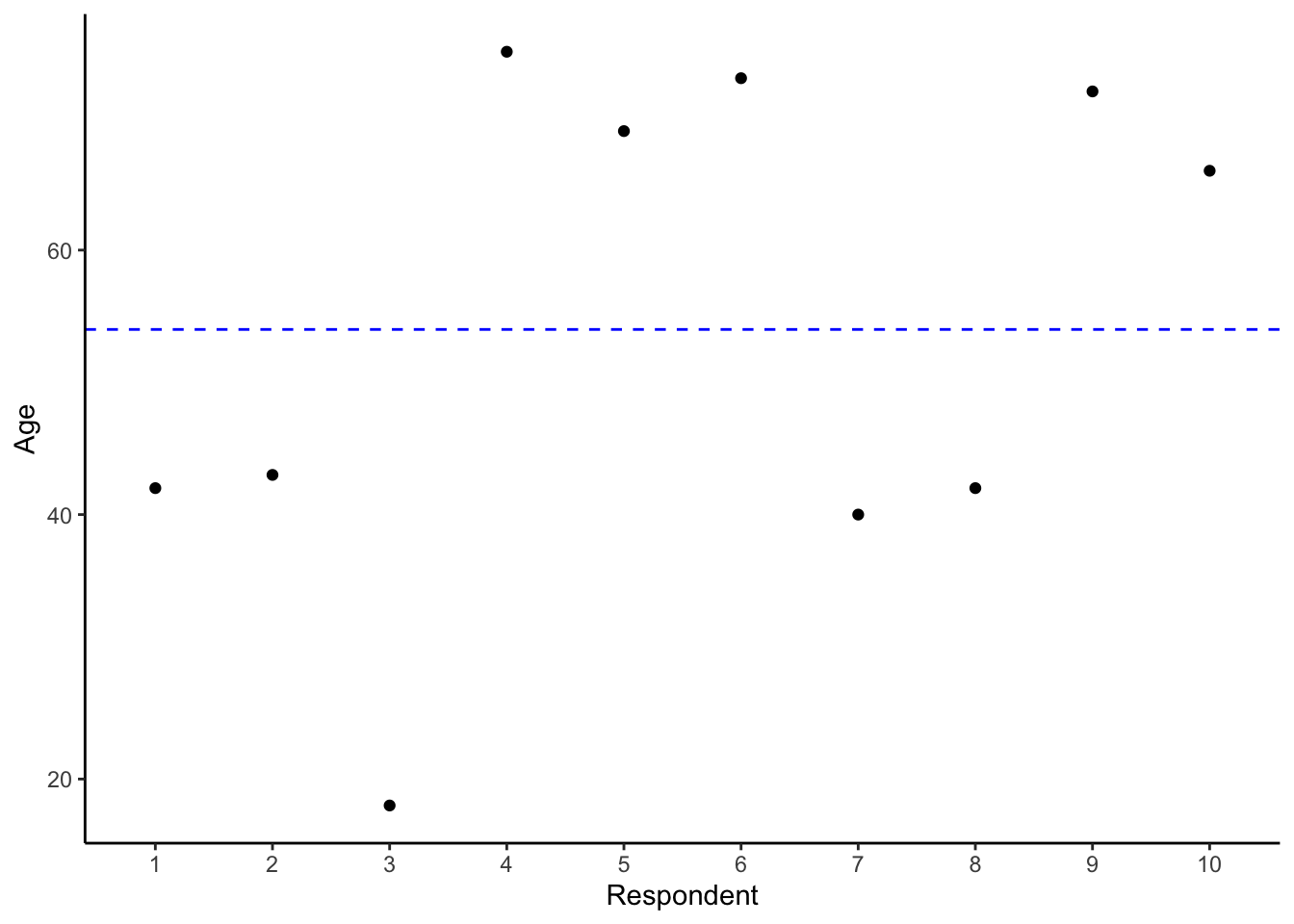Fig.4 Adding the mean age

Then we can add vertical lines measuring the distance (deviation) of each observation from the mean (Fig. 5).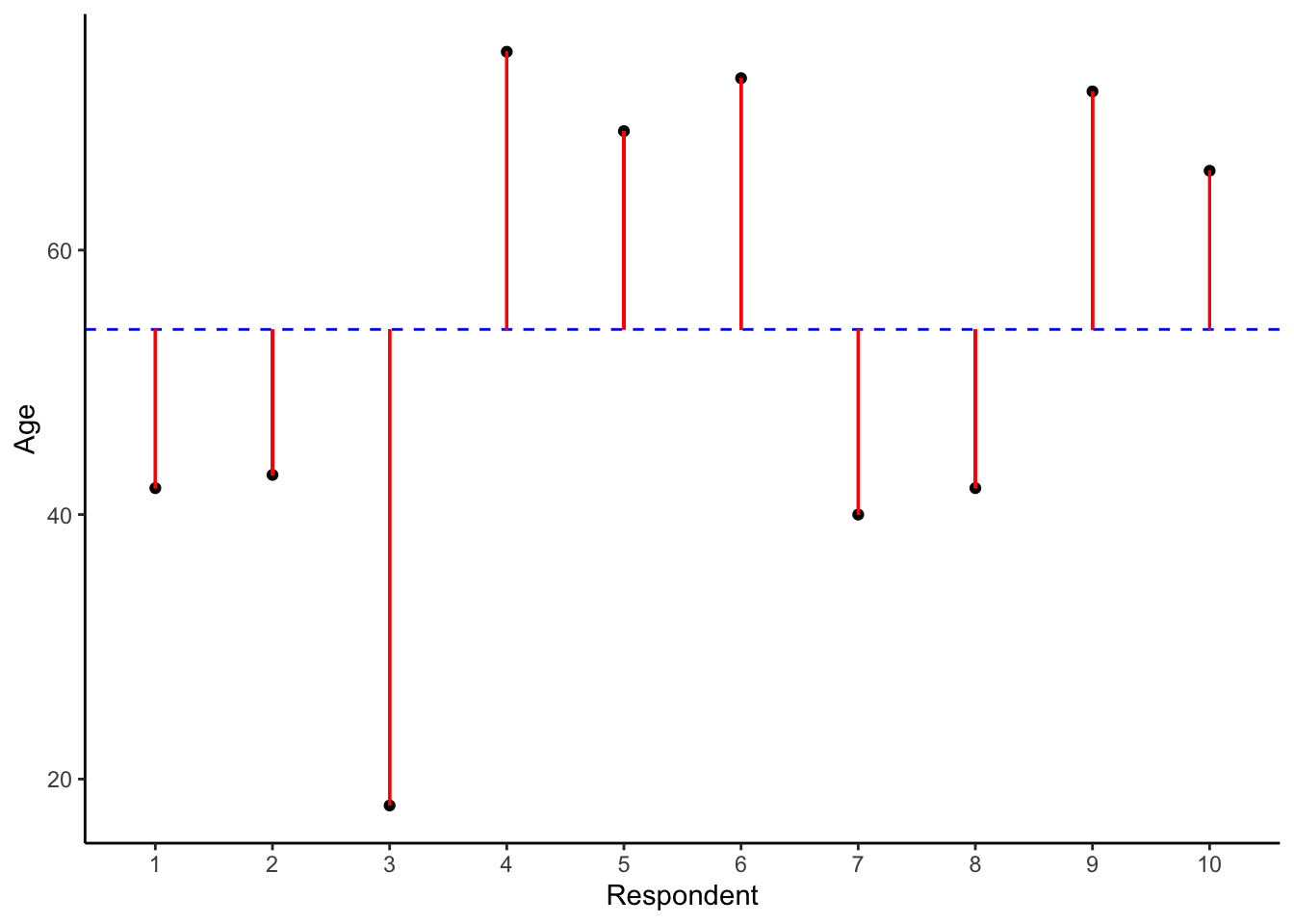Fig.5 Measuring the deviation

### 4.1.2 Model Error

#### 4.1.2.1 Calculating The Error

Clearly, each vertical line in the graph in Fig.5 provides a measure of the difference between one of the observations and the model or mean age.

We can represent this information as a data.frame:

df <- data.frame(Respondent = 1:10,
Age = qlfs.10$Age, model = mean(qlfs.10$Age),
error = qlfs.10$Age - mean(qlfs.10$Age) )
df
Respondent Age model error
1 42 54 -12
2 43 54 -11
3 18 54 -36
4 75 54 21
5 69 54 15
6 73 54 19
7 40 54 -14
8 42 54 -12
9 72 54 18
10 66 54 12

The model we are using to summarise the ages of the population members is the average (central tendency) of the dataset, specifically, the mean age of all members of the population ie. 54.

The difference between the first person’s age and the mean age = 42 - 54 = -12. This difference is known as the model error.

#### 4.1.2.2 Overall Model Error

Looking back to the graph, it is also clear that the greater the total length of the vertical lines (deviations from the model), the worse the model fits the data.

What happens if we change our model from the assumption that everyone is of mean age, to the assumption that everyone is 21? - See Fig.6.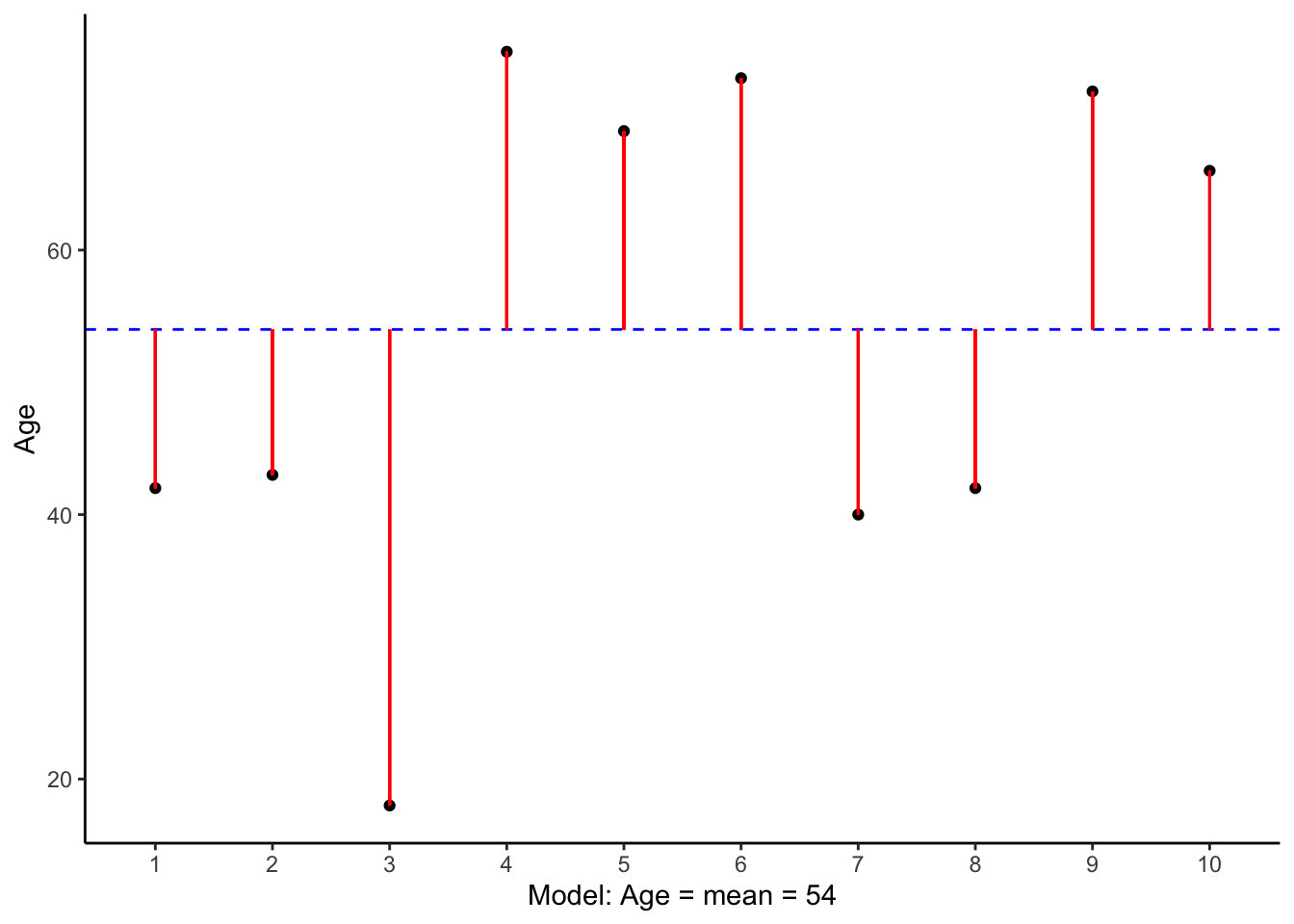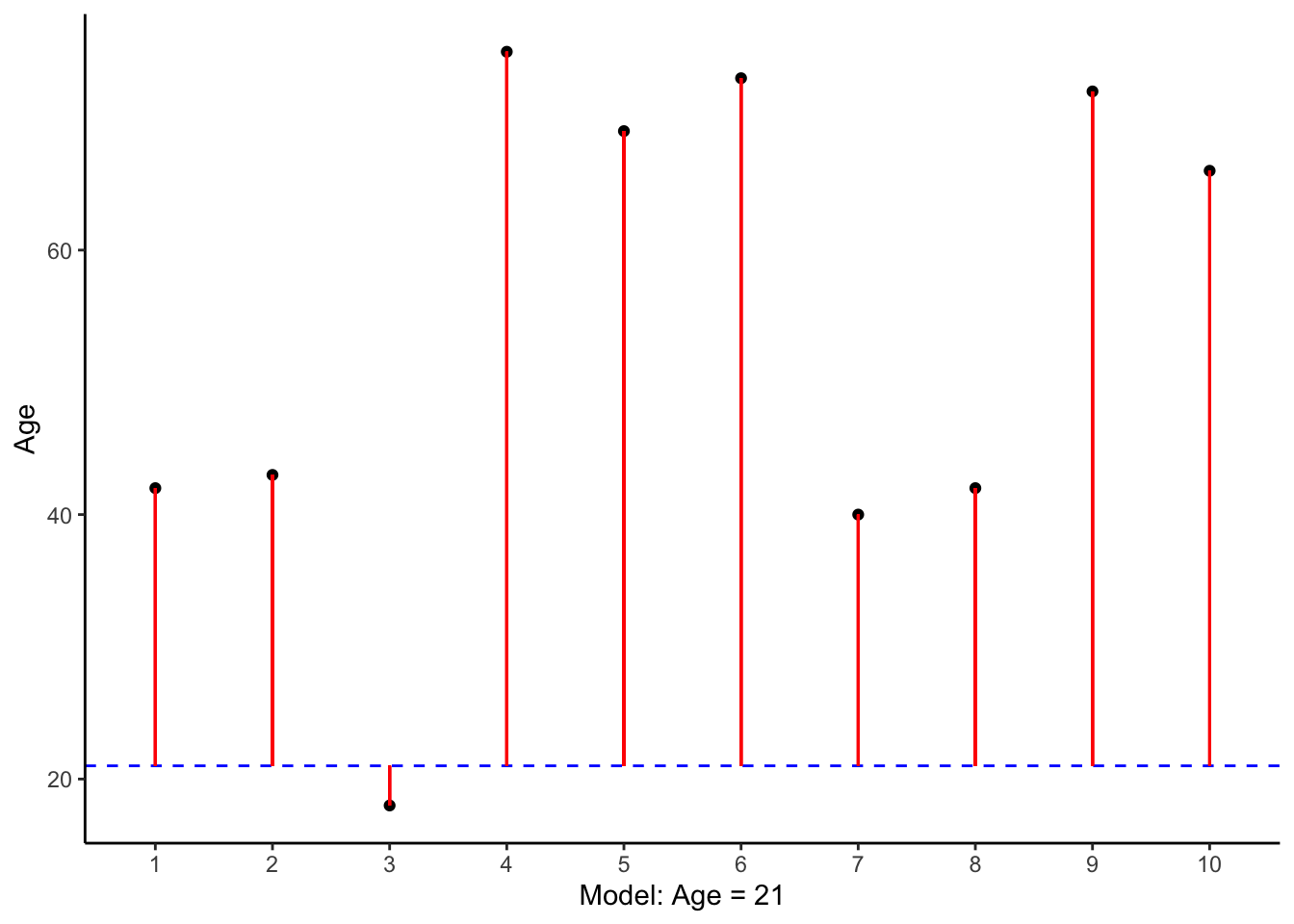Fig.6 Error comparison

The answer is that the total length of the vertical distances from the model is clearly greater when the model is Age = 21 than when the model is Age = 54.

## 4.2 Measures of Dispersion

There are a number of ways of summarising the overall model error.

### 4.2.1 Total Model Error

We can measure the total amount of error by summing up the deviations:

$\mbox{Total Error} = \sum{(x_i - \overline{x}) }$

This is easy to calculate in R using the sum( ) function:

sum(df$error) ##  0 Why was the total error 0? ### 4.2.2 Total Squared Model Error Well, we can square the errors. This works, because a negative times a negative makes a positive. Hence we want to find: $\mbox{Total Squared Error} = \sum{ \left(x_i - \overline{x}\right )^2 }$ Squaring all of the errors gives the following: df$square.error <- df$error^2 df Respondent Age model error square.error 1 42 54 -12 144 2 43 54 -11 121 3 18 54 -36 1296 4 75 54 21 441 5 69 54 15 225 6 73 54 19 361 7 40 54 -14 196 8 42 54 -12 144 9 72 54 18 324 10 66 54 12 144 From which we can derive the total squared error: sum(df$square.error)
##  3396

### 4.2.3 Population Variance

The problem with Total Squared Error is that the larger the number of observations, the more scope there is for model error. Ten observations with a squared error of 0.1 each have a Total Square Error of 10 x 0.1 = 1. Yet one hundred observations, also with a squared error of 0.1 each, will have a larger Total Squared Error because 100 x 0.1 = 10.

Of more interest is the variance: the average (mean) squared error per respondent.

$\mbox{Var} = \sigma^2 = \frac{ \sum{ \left (x_i - \overline{x}\right )^2 } }{N}$

This is easily calculated:

# First count and store the number of observations (rows) in the data.frame
N <- nrow(df)
N
##  10
# Then calculate the variance
variance <- sum(df\$square.error) / N
variance
##  339.6

The larger the dispersion (spread) of the data around the mean, the greater the variance (Fig.7).